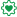# QlikView Scripting

Discussion Board for collaboration on QlikView Scripting.

Announcements
Modernize Your QlikView Deployment webinar, Nov. 3rd. REGISTER
cancel
Showing results for
Did you mean:
HighlightedContributor

## OK LOAD followed by lines of script

OK

Followed by the script that caused the error

Only thing is, there is no error in the script.  The file path is correct, no missing fields in the excel document it's pulling from, etc.

Has anyone else come across this and know why it may be happening or if there is actually an error at all?

14 Replies
HighlightedEmployee

Can you attach a screenshot? Is that error on screen, in the logs? OK LOAD?

Did the script work before? What is that script doing? Is it possible you are loading a field that is not on the source? Can it be that there are two lines with the same field name? Or that the table has been concatenated if it has the same number and name of fields than a previously loaded one?

HighlightedMVP

Can you post a screenshot of the error message?

HighlightedContributorScript wasn't created by me but I am thinking this may be an alert that the script isn't best practice due to the large amount of nested if statements.

HighlightedMVP

Would you also be able to share the script used here?

HighlightedContributor

I removed the confidential info.  See below:

OK

'CNA'                                                                                                                                                                                                                                                                as        StatementCarrier,

Policy                                                                                                                                                                                                                                                                as CommissionStatementPolicyNumber,

[Insured Name]                                                                                                                                                                                                                                                as        Name,

Right(Date([Policy Effective Date],'MM/DD/YYYY'),10)                                                                                                                                                                as        CommissionStatementPolicyEffectiveDate,

([Amount Due CNA]*-1)+[Amount Due Agent]                                                                                                                                                                                        as StatementAgencyCommission,

if(Left([Statement Date],3)='JAN',Right([Statement Date],4)&'01',

if(Left([Statement Date],3)='FEB',Right([Statement Date],4)&'02',

if(Left([Statement Date],3)='MAR',Right([Statement Date],4)&'03',

if(Left([Statement Date],3)='APR',Right([Statement Date],4)&'04',

if(Left([Statement Date],3)='MAY',Right([Statement Date],4)&'05',

if(Left([Statement Date],3)='JUN',Right([Statement Date],4)&'06',

if(Left([Statement Date],3)='JUL',Right([Statement Date],4)&'07',

if(Left([Statement Date],3)='AUG',Right([Statement Date],4)&'08',

if(Left([Statement Date],3)='SEP',Right([Statement Date],4)&'09',

if(Left([Statement Date],3)='OCT',Right([Statement Date],4)&'10',

if(Left([Statement Date],3)='NOV',Right([Statement Date],4)&'11',

if(Left([Statement Date],3)='DEC',Right([Statement Date],4)&'12','REVIEW'))))))))))))   as MonthPaidbyCarrier,

if([Agency Name]='XXX','XXX',

if([Agency Name]='XXX','XXX',

if([Agency Name]='XXX','XXX',

if([Agency Name]=''XXX','XXX',

if([Agency Name]=''XXX','XXX','REVIEW')))))                                                                                                                                as CommissionStatementBranch,

RecNo()                                                                                                                                                                                                                                                                as        RecNo

FROM

(ooxml, embedded labels, table is Sheet1)

HighlightedChampion III

HighlightedMVP

You def. are missing a colon

OK:

'CNA'                                                                                                                                                                                                                                                                as        StatementCarrier,

Policy                                                                                                                                                                                                                                                                as CommissionStatementPolicyNumber,

[Insured Name]                                                                                                                                                                                                                                                as        Name,

Right(Date([Policy Effective Date],'MM/DD/YYYY'),10)                                                                                                                                                                as        CommissionStatementPolicyEffectiveDate,

([Amount Due CNA]*-1)+[Amount Due Agent]                                                                                                                                                                                        as StatementAgencyCommission,

if(Left([Statement Date],3)='JAN',Right([Statement Date],4)&'01',

if(Left([Statement Date],3)='FEB',Right([Statement Date],4)&'02',

if(Left([Statement Date],3)='MAR',Right([Statement Date],4)&'03',

if(Left([Statement Date],3)='APR',Right([Statement Date],4)&'04',

if(Left([Statement Date],3)='MAY',Right([Statement Date],4)&'05',

if(Left([Statement Date],3)='JUN',Right([Statement Date],4)&'06',

if(Left([Statement Date],3)='JUL',Right([Statement Date],4)&'07',

if(Left([Statement Date],3)='AUG',Right([Statement Date],4)&'08',

if(Left([Statement Date],3)='SEP',Right([Statement Date],4)&'09',

if(Left([Statement Date],3)='OCT',Right([Statement Date],4)&'10',

if(Left([Statement Date],3)='NOV',Right([Statement Date],4)&'11',

if(Left([Statement Date],3)='DEC',Right([Statement Date],4)&'12','REVIEW'))))))))))))  as MonthPaidbyCarrier,

if([Agency Name]='XXX','XXX',

if([Agency Name]='XXX','XXX',

if([Agency Name]='XXX','XXX',

if([Agency Name]=''XXX','XXX',

if([Agency Name]=''XXX','XXX','REVIEW')))))                                                                                                                                as CommissionStatementBranch,

RecNo()                                                                                                                                                                                                                                                                as        RecNo

FROM

[\\'XXX','XXX',]

(ooxml, embedded labels, table is Sheet1)

HighlightedSpecialist III

try running the script without the word OK.Contributor

sorry for the confusion.  OK isn't in the script but is in the script error.  It doesn't appear anywhere in the actual script.